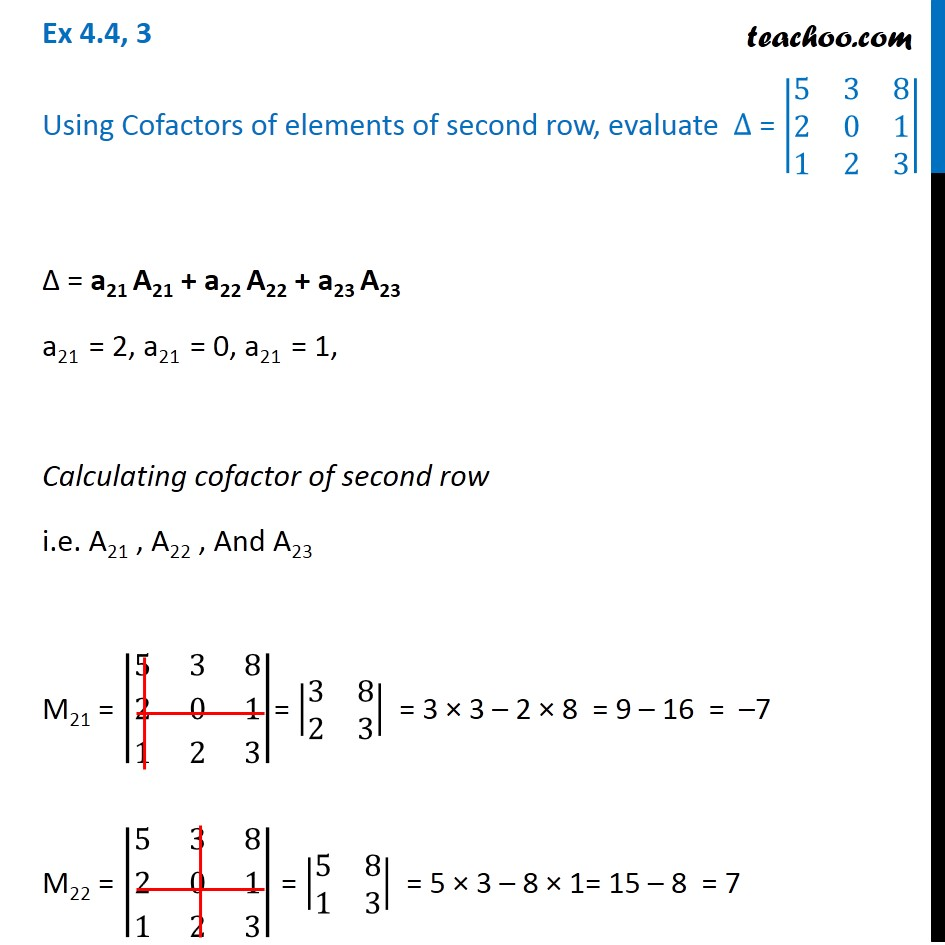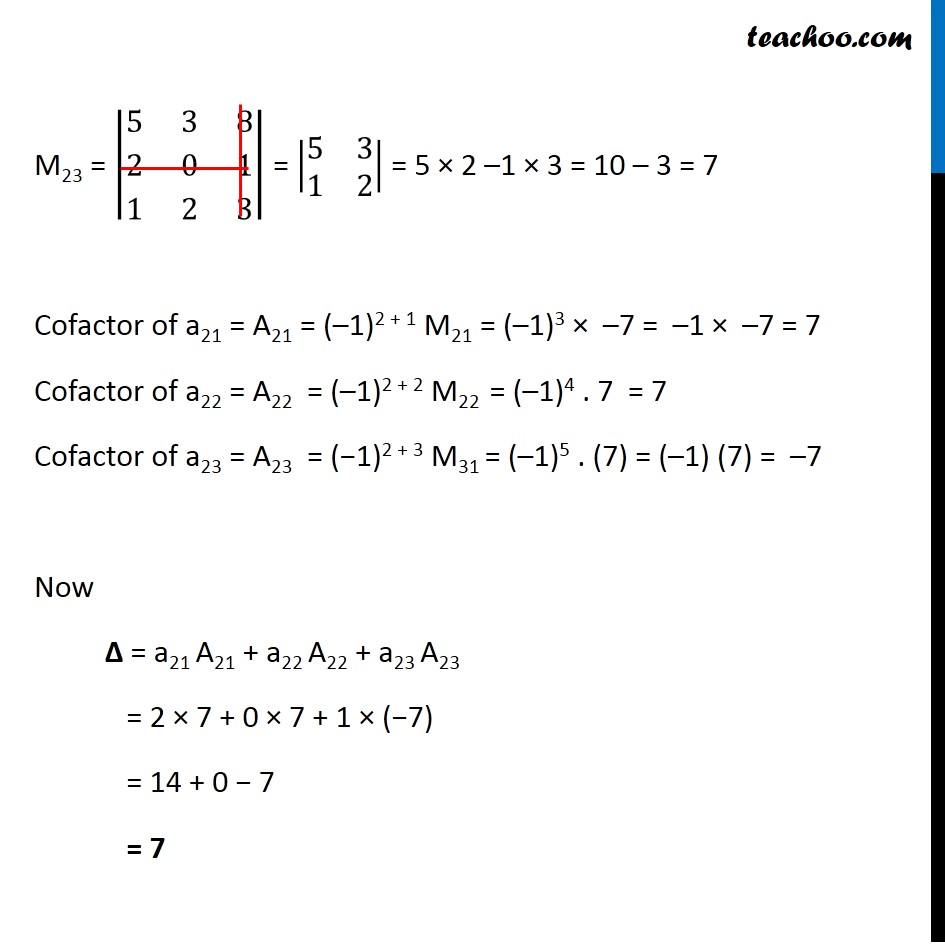Evaluating determinant using minor and co-factor

Chapter 4 Class 12 Determinants
Concept wiseIntroducing your new favourite teacher - Teachoo Black, at only ₹83 per month

### Transcript

Ex 4.4, 3 Using Cofactors of elements of second row, evaluate ∆ = |■8(5&3&8@2&0&1@1&2&3)| Δ = a21 A21 + a22 A22 + a23 A23 a21 = 2, a21 = 0, a21 = 1, Calculating cofactor of second row i.e. A21 , A22 , And A23 M21 = |■8(5&3&8@2&0&1@1&2&3)|= |■8(3&8@2&3)| = 3 × 3 – 2 × 8 = 9 – 16 = –7 M22 = |■8(5&3&8@2&0&1@1&2&3)| = |■8(5&8@1&3)| = 5 × 3 – 8 × 1= 15 – 8 = 7 M23 = |■8(5&3&8@2&0&1@1&2&3)| = |■8(5&3@1&2)| = 5 × 2 –1 × 3 = 10 – 3 = 7 Cofactor of a21 = A21 = (–1)2 + 1 M21 = (–1)3 × –7 = –1 × –7 = 7 Cofactor of a22 = A22 = (–1)2 + 2 M22 = (–1)4 . 7 = 7 Cofactor of a23 = A23 = (−1)2 + 3 M31 = (–1)5 . (7) = (–1) (7) = –7 Now Δ = a21 A21 + a22 A22 + a23 A23 = 2 × 7 + 0 × 7 + 1 × (−7) = 14 + 0 − 7 = 7